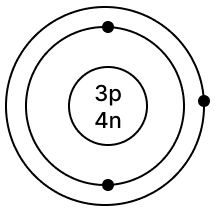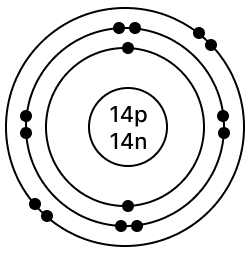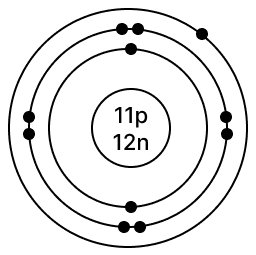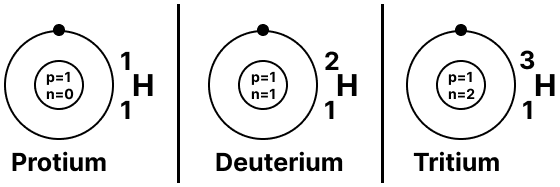# Atomic Structure

## The atom - ancient views

#### Question 1

State the main postulates of - Dalton's atomic theory. Explain how the modern atomic theory contradicted Dalton's atomic theory.

Postulates of Dalton's atomic theory : Matter consists of particles called - atoms, which are indivisible & cannot be created or destroyed.

1. Atoms of the same element are alike but differ from atoms of all other elements.
2. Compounds or molecules are formed when atoms combine with other atoms in simple whole number ratios.
3. An atom is the smallest unit of matter which takes part in a chemical reaction.

Modern atomic theoryDalton's atomic theory
Atoms are destructibleAtoms are indestructible
Atoms are divisible & consist of - protons, electrons & neutronsAtoms are indivisible & smallest particle of an element.
Atoms of the same element may not be alike as seen in case of isotopesAtoms of the same element are alike in all respect.

## Sub-atomic particles

#### Question 2

With reference to the discovery of the structure of an atom, explain in brief - William Crookes experiment for the discovery of cathode rays, followed by - J.J. Thomsons experiment pertaining to the constituents of the cathode rays.
State which sub-atomic particle was discovered from his experiment.

William Crookes Experiment:

William Crookes passed an electric discharge through a discharge tube containing a gas at low pressure. Blue rays were emitted from the cathode [negative plate] which were called cathode rays.

J.J. Thompson's Experiment:

In his experiments, J.J. Thomson studied the characteristics and constituents of cathode rays and observed that the rays have kinetic energy & cast a shadow of an object in their path. They were deflected by a positively charged plate.

Discovery of electron:

This proved that the cathode rays consisted of negatively charged sub-atomic particles called electrons.

#### Question 3

Explain in brief - Goldstein's experiment which led to the discovery of the proton and - Lord Rutherford's experiment which led to the discovery of the atomic nucleus.

Goldstein's Experiment:

Goldstein used a modified cathode ray tube with a perforated cathode and observed new type of rays produced from the anode passing through the hole of the perforated cathode. These rays were called - Anode rays.

The positive rays were affected by electric & magnetic fields but in a direction opposite to that of cathode rays.

Goldstein concluded that anode rays or positive rays consist of positively charged particles called protons.

Lord Rutherford's Experiment:

Lord Rutherford projected alpha particles towards a thin gold foil in the path of the rays.

He observed that most of the alpha particles went straight through the foil but some were deflected slightly & some by large angles.

He concluded that an atom on the whole is relatively empty but consists of a concentrated positive mass in the centre which lead to the deflection of the alpha particles. Hence, Lord Rutherford discovered atomic nucleus.

#### Question 4

'Electrons revolve around the nucleus in fixed orbits or shells called energy levels'. State how these energy levels are represented.

The energy levels 1,2,3... are represented by integer 'n' or as K, L, M, N...

#### Question 5

Draw a neat labeled diagram representing an atom. Name the three sub-atomic particles in the atom & represent them symbolically showing the mass & charge of each. State where the sub-atomic particles are present in the atom.

Below labelled diagram shows the representation of an atom as per the modern atomic theory: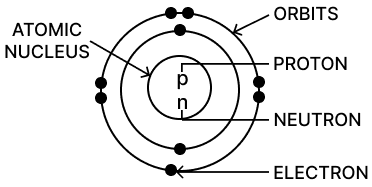The three sub-atomic particles in the atom are:

S.
No.
Sub-atomic
particle
Symbol
1.Proton+1p1
2.Neutron0n1
3.Electron-1e0

Protons and neutrons are found inside the nucleus of the atom. Electrons are distributed across energy levels outside of the nucleus.

## Terms

#### Question 6

Define the term - 'atomic number' of an atom. If an atom 'A' has an atomic number of - eleven, state the number of protons & electrons it contains.

Atomic number of an atom is equal to number of protons in the nucleus of an atom. As number of electrons = number of protons hence, atomic number is also equal to the number of electrons in an element.

If atomic number [Z] is 11, the number of electrons = number of protons = 11.

#### Question 7

Define the term - 'mass number' of an atom. If an atom 'B' has mass number 35 & atomic number 17, state the number of protons, electrons & neutrons it contains.

Mass number is equal to the total number of protons & neutrons in the atom of an element.

If,
mass number [A] = 35,
atomic number [Z] = 17 then
number of protons = number of electrons = 17
number of neutrons [n] = A - Z = 35 - 17 = 18 neutrons

#### Question 8

State why the atomic weight of an element is also termed - relative atomic mass.

As atomic weight is the number of times, one atom of an element is heavier than the mass of an atom of hydrogen or 1⁄12 the mass of an atom of carbon, hence, it is also termed as Relative Atomic Mass.

#### Question 9

State how electrons are distributed in an atom. Explain in brief the rules which govern their distribution.

Electrons revolve around the nucleus in imaginary paths called orbits or shells. Different Orbits are K shell [first shell, n=1], L shell [second shell, n=2] M, N...... etc.

Rules governing the distribution of electrons are summarized below:

1. The maximum number of electrons in each shell or orbit is determined by the formula 2n2, where n is the number of shell.
K shell, n=1, no. of electrons = 2 x 12 = 2
L shell, n=2, no. of electrons = 2 x 22 = 8
M shell, n=3, no. of electrons = 2 x 32 = 18
2. Electrons are not accommodated in a given shell, unless the inner shells are filled. Shells are filled in step-wise manner.
3. The atom's outermost shell needs 2 electrons for stability (duplet rule) if it has only one shell. If it has multiple shells, it requires 8 electrons (octet rule) in the outermost shell for stability.

#### Question 10

If an atom 'A' has atomic number 19 & number 39, state -

(i) Its electronic configuration.

(ii) The number of valence electrons it possesses.

(i) As atomic number [Z] is 19 so electronic configuration = [2,8,8,1]

(ii) Number of valence electrons = 1

## Arrangement of electrons

#### Question 11

Draw the atomic diagrams of the following elements showing the distribution of - protons, neutrons & the electrons in the various shells of the atoms.

(a) Carbon - 126C

(b) Oxygen - 168O

(c) Phosphorus - 3115P

(d) Argon - 4018Ar

(e) Calcium - 4020Ca

[The upper number represents the - mass number & the lower number the - atomic number e.g. calcium - mass number = 40, atomic number = 20]

(a) Carbon - 126C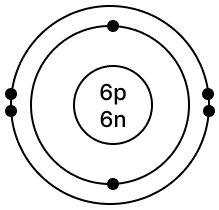(b) Oxygen - 168O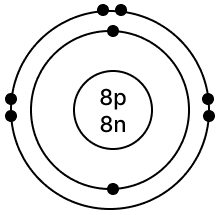(c) Phosphorus - 3115P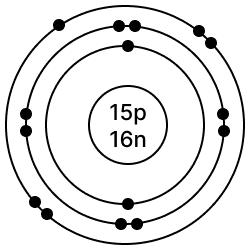(d) Argon - 4018Ar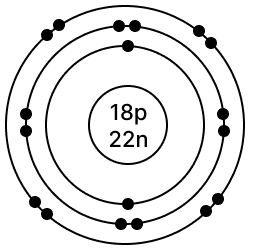(e) Calcium - 4020Ca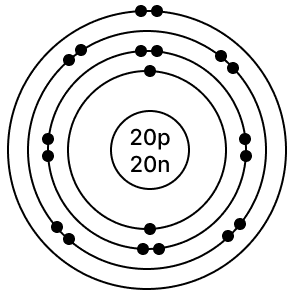## Valency

#### Question 12

'Valency' is the number of hydrogen atoms which can combine with [or displace] one atom of the element [or radical] forming a compound. With reference to the above definition of valency, state the valency of chlorine in hydrogen chloride, giving reasons.

In hydrogen chloride, one atom of hydrogen combines with one atom of chlorine so valency of chlorine in hydrogen chloride is 1.

#### Question 13

'Valency' is also the number of electrons - donated or accepted by an atom so as to achieve stable electronic configuration of the nearest noble gas'. With reference to this definition -

(a) State what is meant by 'stable electronic configuration'.

(b) State why the valency of -

(i) sodium, magnesium & aluminium is: +1, +2 & +3 respectively.

(ii) chlorine, oxygen & nitrogen is: -1, -2 & -3 respectively.

(a) Stable electronic configuration — The atom's outermost shell needs 2 electrons for stability (duplet rule) if it has only one shell. If it has multiple shells, it requires 8 electrons (octet rule) in the outermost shell for stability.

(b) (i) The atoms of sodium, magnesium and aluminium donate 1, 2 and 3 electrons, respectively, in order to achieve a stable electronic configuration of 8 electrons (octet rule) in the outermost shell. Hence, their valencies are +1, +2 & +3.

(ii) The atoms of chlorine, oxygen and nitrogen accept 1, 2 and 3 electrons, respectively, in order to achieve a stable electronic configuration of 8 electrons (octet rule) in the outermost shell. Hence, their valencies are -1, -2 & -3.

## Formation of compounds

#### Question 14

With reference to formation of compounds from atoms by electron transfer - electrovalency, state the basic steps in the conversion of sodium & chlorine atoms to sodium & chloride ions leading to the formation of the compound - sodium chloride.

[electronic configuration of: Na = 2,8,1 & Cl = 2,8,7]

Formation of compounds from atoms by electron transfer

• Electronic configuration of Na = 2,8,1

• Nearest noble gas = Neon [2,8]

• Na loses one electron from outermost shell to attain stability

• Electronic configuration of Cl = 2,8,7

• Nearest noble gas = Argon [2,8,8]

• Cl gains one electron in outermost shell to attain stability.

Hence, the electron given by Na is gained by Cl, both attain a stable configuration and sodium chloride is formed.

## Objective Type Questions

#### Question 1

Match the statements in List I with the correct answer from List II.

List IList II
1. Mass number of an atom is the number of protons andA: Electron
2. The sub-atomic particle with a negligible mass.B: Argon
3. An atom having stable electronic configuration.C: Nitrogen
4. A molecule formed by sharing of electrons [covalency].D: Sodium
5. A metallic atom having unstable electronic configuration.E: Neutrons

List IList II
1. Mass number of an atom is the number of protons andE: Neutrons
2. The sub-atomic particle with a negligible mass.A: Electron
3. An atom having stable electronic configuration.B: Argon
4. A molecule formed by sharing of electrons [covalency].C: Nitrogen
5. A metallic atom having unstable electronic configuration.D: Sodium

#### Question 2

Select the correct answer from the choice in bracket to complete each sentence:-

1. An element 'X' has six electrons in its outer or valence shell. Its valency is ............... [+2/-2/-1].

2. An element 'Y' has electronic configuration 2, 8, 6. The element 'Y' is a ............... [metal/non-metal/noble gas].

3. A ............... [proton/neutron] is a sub-atomic particle with no charge and unit mass.

4. An element Z with zero valency is a ............... [metal/noble gas/non-metal].

5. Magnesium atom with electronic configuration 2, 8, 2 achieves stable electronic configuration by losing two electrons, thereby achieving stable electronic configuration of the nearest noble gas ............... [neon/argon].

1. An element 'X' has six electrons in its outer or valence shell. Its valency is -2.

2. An element 'Y' has electronic configuration 2, 8, 6. The element 'Y' is a non-metal.

3. A neutron is a sub-atomic particle with no charge and unit mass.

4. An element Z with zero valency is a noble gas.

5. Magnesium atom with electronic configuration 2, 8, 2 achieves stable electronic configuration by losing two electrons, thereby achieving stable electronic configuration of the nearest noble gas neon.

#### Question 3

The diagram represents an isotope of hydrogen [H]. Answer the following: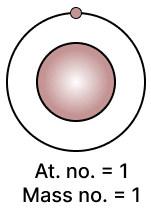1. Are isotopes atoms of the same element or different elements.

2. Do isotopes have the same atomic number or the same mass number.

3. If an isotope of 'H' has mass no. = 2, how many electrons does it have.

4. If an isotope of 'H' has mass no. = 3, how many neutrons does it have.

5. Which sub-atomic particles in the 3 isotopes of 'H' are the same.

1. Isotopes are atoms of the same element.

2. Isotopes have same atomic number.

3. Number of electrons in an isotope of 'H' having mass no. = 2 is 1 as its atomic number is 1.

4. Number of neutrons in an isotope of 'H' having mass no. [A] = 3 is 2, because, atomic number [Z] is 1 so number of protons = number of electrons = 1 and number of neutrons [n] = A - Z = 3 - 1 = 2.

5. Number of electrons and protons are same in the 3 isotopes of 'H' as atomic number is the same.

#### Question 4

State the electronic configuration for each of the following:

1. Hydrogen [p = 1].

2. Boron [p = 5]

3. Nitrogen [p = 7].

4. Neon [p = 10]

5. Magnesium [p = 12].

6. Aluminium [p = 13].

7. Sulphur [p = 16].

8. Argon [p = 18].

9. Potassium [p = 19].

10. Calcium [p = 20].

S.
No.
ElementElectronic
Configuration
1.Hydrogen [p = 1]1
2.Boron [p = 5]2, 3
3.Nitrogen [p = 7]2, 5
4.Neon [p = 10]2, 8
5.Magnesium [p = 12]2, 8, 2
6.Aluminium [p = 13]2, 8, 3
7.Sulphur [p = 16]2, 8, 6
8.Argon [p = 18]2, 8, 8
9.Potassium [p = 19]2, 8, 8, 1
10.Calcium [p = 20]2, 8, 8, 2

#### Question 5

Draw the structure of the following atoms showing the nucleus containing - protons, neutrons and the orbits with the respective electrons:

1. Lithium [At. no. = 3, Mass no. = 7].

2. Carbon [At. no. = 6, Mass no. = 12].

3. Silicon [At. no. 14, Mass no. = 28].

4. Sodium [At. no. = 11, Mass no. = 23].

5. Isotopes of hydrogen [11H, 12H, 13H]